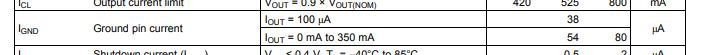If you have a related question, please click the "Ask a related question" button in the top right corner. The newly created question will be automatically linked to this question.

# TPS720: # QA78A60 - Efficiency of P/N: TPS72025QDRVRQ1 - regulation of voltage output

Part Number: TPS720

Hello,

can you please advise the efficiency level of the voltage regulator P/N: TPS72025QDRVRQ1 and if/how the output voltage can be regulated?

Or would there be another high efficiency voltage regulator available with an even higher efficiency level?

Required a high efficiency voltage regulator with an input voltage of 4.5VDC and an output voltage of 2.5VDC

Thank you.

Best regards

The efficiency for an LDO depends on the application conditions itself.  We can calculate your application's efficiency (n) using the following equation:

n = (Pout / Pin) x 100

n = ([Vout x Iout] / [Vin x Iin]) x 100

n = ([2.5 V x Iout] / [4.5 V x (Iout + Ignd)]) x 100

At this point we will assume that your Iout is 100 mA for this example.  You can adjust based on your actual application.  Ignd comes from the datasheet:n = ([2.5 V x 100 mA] / [4.5 V x (100 mA + 54 uA)]) x 100

n = ([2.5 V x 100 mA] / [4.5 V x 100.054 mA]) x 100

n = (250 mW / 450.243 mW) x 100

n = 55.526%

Commonly you will see this calculation simplified for LDOs when Iout >> Ignd to

n = (Vout / Vin) x 100

n = (2.5 V / 4.5 V) x 100

n = 55.556%

Using the simplified efficiency equation, you can see that the smaller the headroom (Vin - Vout) is in the application, the more efficient the application will be.  The minimum headroom (Vin - Vout) for an LDO to be able to regulate the output voltage is equal to the LDO's dropout (Vdo).  As such, the LDO will be most efficient (consume the least amount of power) when Vin is equal to Vout + Vdo.

TPS720 has a maximum Vin dropout of 200 mV.  As such, you can increase the efficiency in your application by reducing the input voltage closer to 2.7 V.  The most efficient way to do this is by using a switching regulator.

Very Respectfully,

Ryan